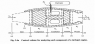# Aeroengines/Fluid Mechanics - Steady Flow

• JRS192
In summary, the conversation discussed confusion between the universal gravitational constant G and the acceleration due to gravity on earth, gc. The question was about determining V9 using given values, but there was a discrepancy in the answer due to confusion between these two constants. The speaker researched and used gc instead of G, resulting in an incorrect answer. The expert clarified the distinction between the two constants and their uses in different types of calculations.

## Homework Statement

See Fig.2.4a, let the gases flowing through the nozzle control volume be perfect with Cp = 1.004kJ/kg.K

Determine V9 if T5 = 1000K, T9 = 670K and V5 = 120ms^-1.

## Homework Equations

I've detailed the equations in the attached pdf for clarity.

## The Attempt at a Solution

See attached pdf. The question paper has the answer as 822.8m/s, but I'm consistently getting 120m/s. Where am I going wrong?

Thank you

#### Attachments

•Fig2.4a.png
47.3 KB · Views: 459
• doc00586420150126151206.pdf
329.4 KB · Views: 185
You've confused the universal gravitational constant G for the acceleration due to gravity on earth, gc.
It's not clear why you don't understand the distinction between these two constants.

Hi SteamKing,

Thanks for that. I did some research and everything directed me to that figure, even to the point of using gc...

JRS192 said:
Hi SteamKing,

Thanks for that. I did some research and everything directed me to that figure, even to the point of using gc...

I doubt that. You have misinterpreted something in what you read.

The universal gravitational constant G (which is invariably capitalized) is typically used to find the force of gravitational attraction between two masses, as described by the formula

F = Gm1m2/r2

G is used more often in physics type calculations. G also has some rather unusual units: G = 6.67*10-11 m3/kg-s2

The acceleration due to gravity on earth, gc, which is invariably lower case, is typically commonly found in engineering type calculations, more so than G.